output.to from Sideway
Mechanics: Statics

Forces in Space

1

Draft for Information Only

# Forces in Space

In practical applications, forces are usually involved in three dimensional space. Forces in a plane is only a special three dimensional case with the third direction equals to zero.

Force Decomposition

A force can be represented by a force vector, F, in three dimensional space. The force can be regarded as a plane force by assuming a plane passing through the force vector and normal to coordinate plane zx.  And therefore force, F can be decomposed into a force, Fy along y axis and a force, Fzx in plane zx. Similarly, force, Fzx in plane zx can further be decomposed  into a force, Fx along x axis and a force, Fz along z axis.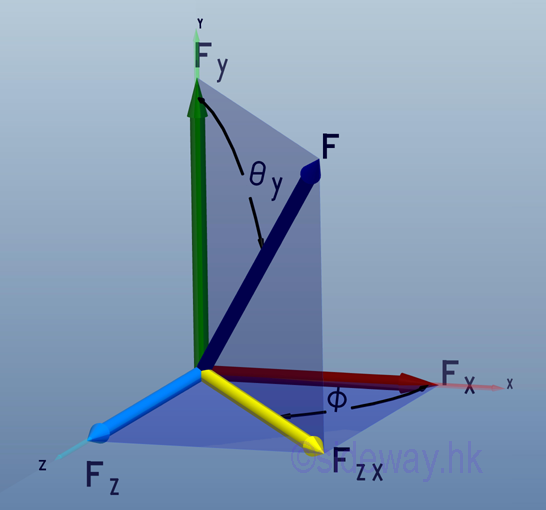Therefore force vector, F, in three dimensional space can be decomposed into Fx, Fy, and Fz. Applying the laws of trigonometric, the magnitude of the three rectangular force vector components are: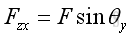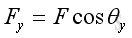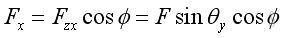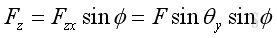And the magnitude of force vector, F, can be obtained by applying the Pythagorean theorem, imply: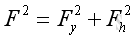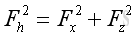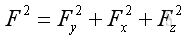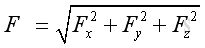The resultant force vector, F, can be obtained by vector addition of the three rectangular force vector components by applying Parallelogram Law, imply: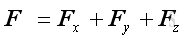Assuming three unit vectors, i, j, and k, with unit magnitude along the x, y, and z axis. then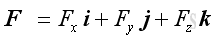ImplyThe three rectangular force vector components  of the resultant force vector, F, can be arranged in a box, imply: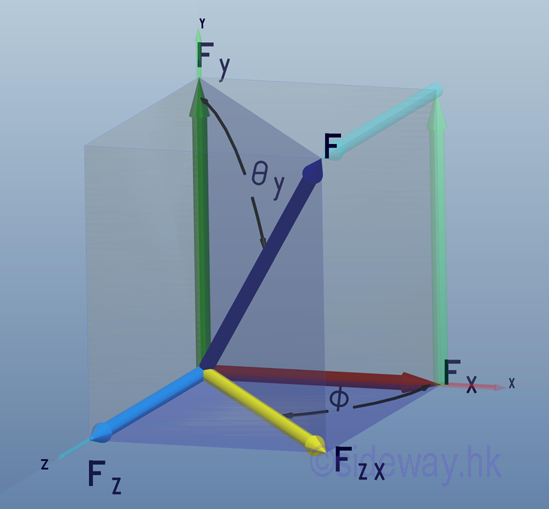Direction Cosines

The direction of the force vector, F can be defined by the angles formed by the force vector and the three coordinate axes, θx,  θy, and θz. Imply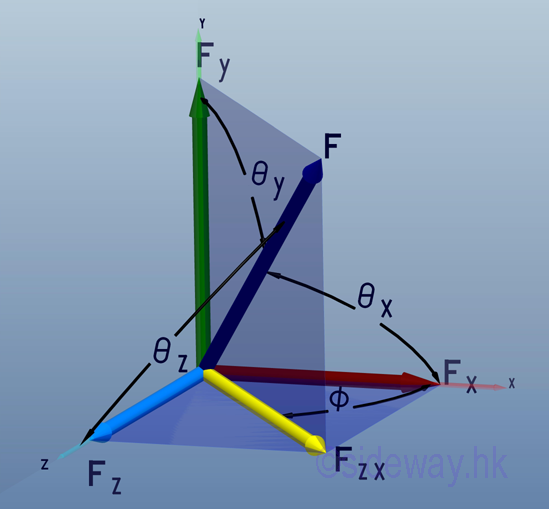The magnitude of the three rectangular force vector components can be defined as: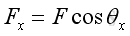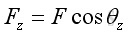The cosine of θx, θy, and θz are known as the direction cosines of the force vector, F. And the force vector becomes two dimensional force when one of the direction cosines equal to zero, that is angle equals 90 degree.

The magnitude of force vector, F can therefore be expressed in terms of  direction cosines as following: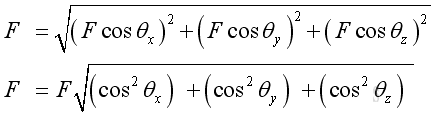Imply the sum of the squares of direction consines equals to 1, that is: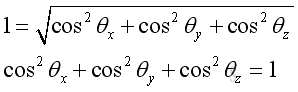And the values of direction consines or the angles are not independent.

The force vector, F can also be expressed in terms of unit coordinate vectors and direction cosines as following: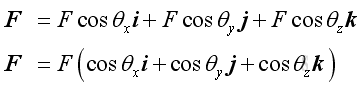The format of the force vector expression can be treated as the product of the force magnitude and the unit direction vector. Let λ be the unit direction vector. Then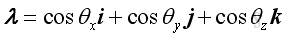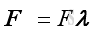Similarly, the unit direction vector can also decomposed to three rectangular vectors: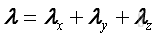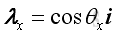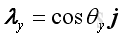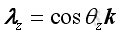The magnitude of the unit direction vector equals to one because: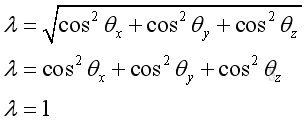The relationship of force vectors and  unit vectors is: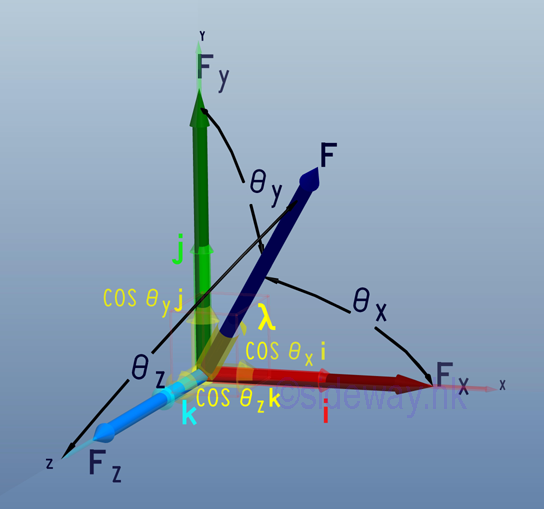Laws of Trigonometric Relations

The direction of a force in space along the line of action can also be respresented by a relative position vector, d.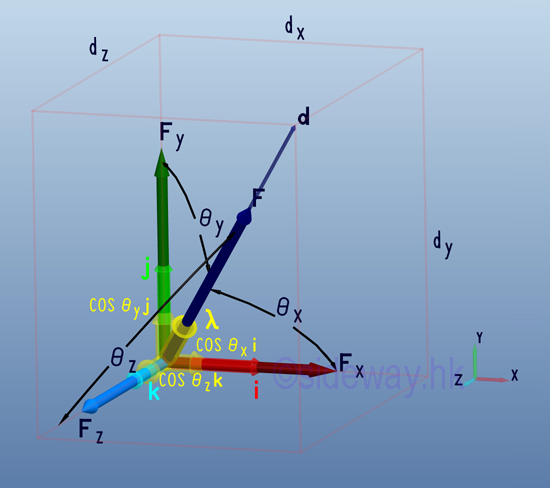Similar to the force vector, F, the relative position vector, d can be expressed as: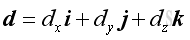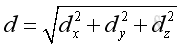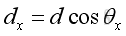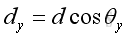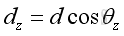Applying the laws of trigonometric relations, imply: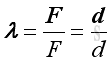And: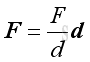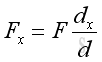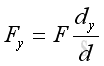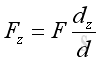©sidewayReferences

1. I.C. Jong; B.G. rogers, 1991, Engineering Mechanics: Statics and Dynamics, Saunders College Publishing, United States of America
2. F.P. Beer; E.R. Johnston,Jr.; E.R. Eisenberg, 2004, Vector Mechanics for Engineers: Statics, McGraw-Hill Companies, Inc., New YorkID: 110400005 Last Updated: 6/23/2013 Revision: 1 Ref:Home (5)

Business

Management

HBR (3)

Information

Recreation

Hobbies (7)

Culture

Chinese (1097)

English (336)

Reference (66)

Computer

Hardware (149)

Software

Application (187)

Digitization (24)

Numeric (19)

Programming

Web (648)CSS (SC)

ASP.NET (SC)

HTML

Knowledge Base

Common Color (SC)

Html 401 Special (SC)

OS (389)

MS Windows

Windows10 (SC)

.NET Framework (SC)

DeskTop (7)

Knowledge

Mathematics

Formulas (8)

Number Theory (206)

Algebra (20)

Trigonometry (18)

Geometry (18)

Calculus (67)

Complex Analysis (21)

Engineering

Tables (8)

Mechanical

Mechanics (1)

Rigid Bodies

Statics (92)

Dynamics (37)

Fluid (5)

Control

Acoustics (19)

Biology (1)

Geography (1)

Copyright © 2000-2019 Sideway . All rights reserved Disclaimers last modified on 10 Feb 2019